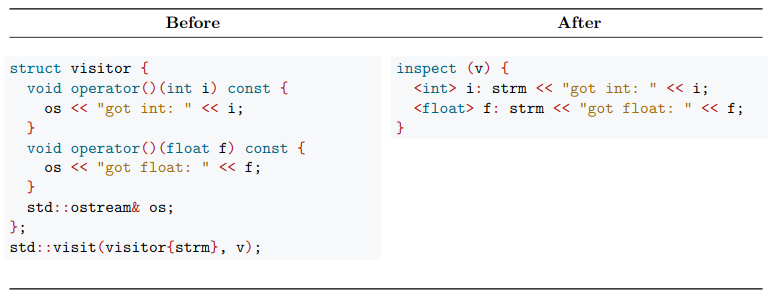# TL;DR

## Python and C++An example from a C++ evolution proposal.

# Pattern matching in Rust

`#[derive(Hash, Debug, PartialEq, Eq, PartialOrd, Ord)] /* A */pub enum Category {  Cute,  Weird,  Endangered,}`
`fn cat_species(v: &str) -> Category {  match v {    "aye-aye" => Category::Endangered, /* A */    _ => Category::Cute, /* B */  }}`
`fn cat_animal_first_attempt(v: &Value) -> Category {  match v["coat"].as_str() {    Some("fur") | Some("feathers") => Category::Cute,    _ => Category::Weird,  }}`
`fn cat_animal_first_attempt_1(v: &Value) -> Category {  let cat = match v["coat"].as_str() { /* A */    Some("fur") | Some("feathers") => Category::Cute, /* B */    _ => Category::Weird,  }  match v["mutation"].as_str() {    Some("albino") => Category::Endangered,    _ => cat  }}`
`fn cat_animal(v: &Value) -> Category {  if let Some("albino") = v["mutation"].as_str() {    Category::Endangered  } else if let Some("fur")              | Some("feathers")              = v["coat"].as_str() {    Category::Cute  } else {    Category::Weird  }`

# Putting it all together

`pub fn categorise(  data: HashMap<String, Vec<Value>>,) -> HashMap<Category, Vec<String>> {  let mut retval = HashMap::new();  for (species, animals) in data {    for animal in animals {          if let Some(name) = (animal["name"].as_str()) { /* A */        retval          .entry(max(cat_species(species.as_str()),                     cat_animal(&animal))) /* B */          .or_insert(Vec::new()) /* C */          .push(name.to_string())      }          }  }  retval}`

# Patterns in modern JavaScript

`const foldAndDump = (path, xs, ...cutoffs) => {  // snip  for (c of cutoffs) {    //snap  }}`
`var rs = [];for (let [printing, info] of     Object.entries(allPrintingsJson['data'])){    rs.push({ ...info, "_pv_set": printing });}`
`const xs = [1,2,3];console.log(sum(...xs));`
`> [a,b] = [1,2][ 1, 2 ]> {x,y} = {y: a, x: b}{ y: 1, x: 2 }> {k,l} = {y: a, x: b}{ y: 1, x: 2 }> [a,b,x,y,k,l][ 1, 2, 2, 1, undefined, undefined ]`

# Packing and unpacking in Python

`>>> a, *b, c = {'hello': 'world', 4: 2, 'rest': True, False: False} >>> a, b, c ('hello', [4, 'rest'], False)`
`>>> print(*[1, 2, 3])1 2 3`
`>>> print({'x': True, **{'y': False}, **{'x': False, 'z': True}}) {'x': False, 'y': False, 'z': True}`

# Pack your bags: we’re going pattern matching

## Pattern matching in JavaScript

Educate, explore and empower. Concisely. Doma.dev